Скачать презентацию Physics of Semiconductor Devices Lecture 2. Carrier

lecture_2.pptx

• Размер: 1.5 Мб
• Автор:
• Количество слайдов: 25

## Описание презентации Physics of Semiconductor Devices Lecture 2. Carrier по слайдам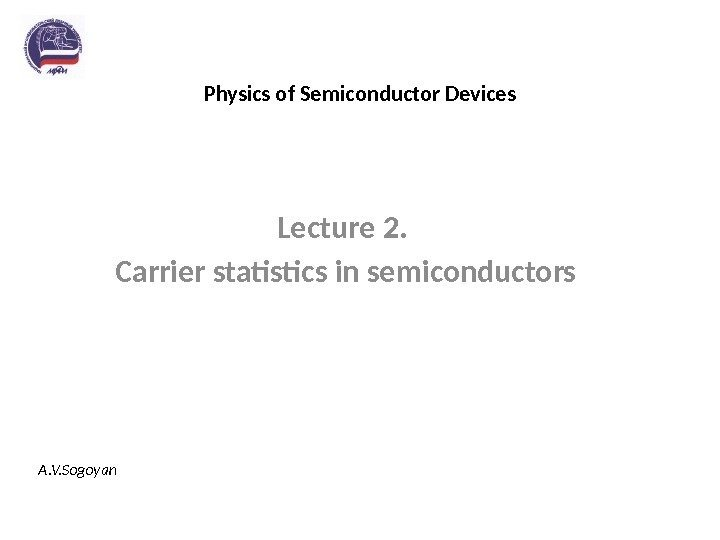Physics of Semiconductor Devices Lecture 2. Carrier statistics in semiconductors A. V. Sogoyan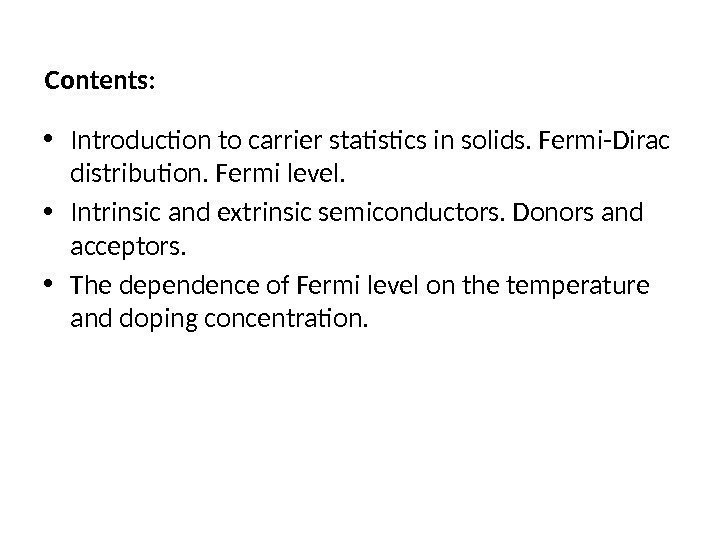Contents: • Introduction to carrier statistics in solids. Fermi-Dirac distribution. Fermi level. • Intrinsic and extrinsic semiconductors. Donors and acceptors. • The dependence of Fermi level on the temperature and doping concentration.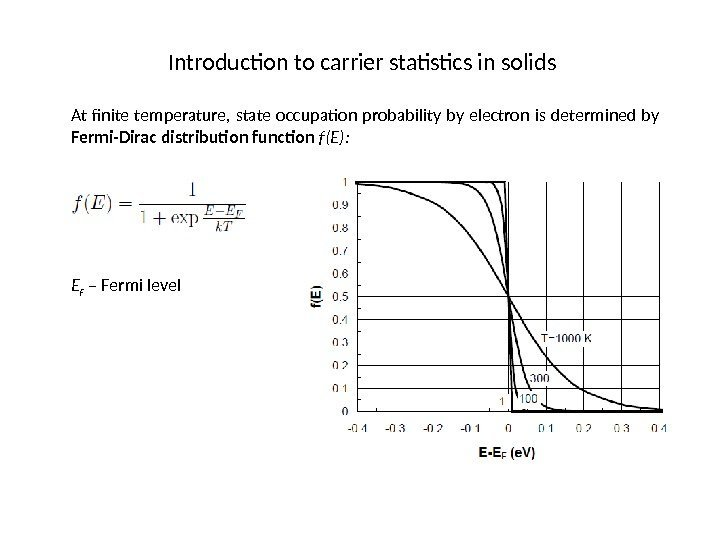Introduction to carrier statistics in solids At finite temperature, state occupation probability by electron is determined by Fermi-Dirac distribution function f(E): E F – Fermi level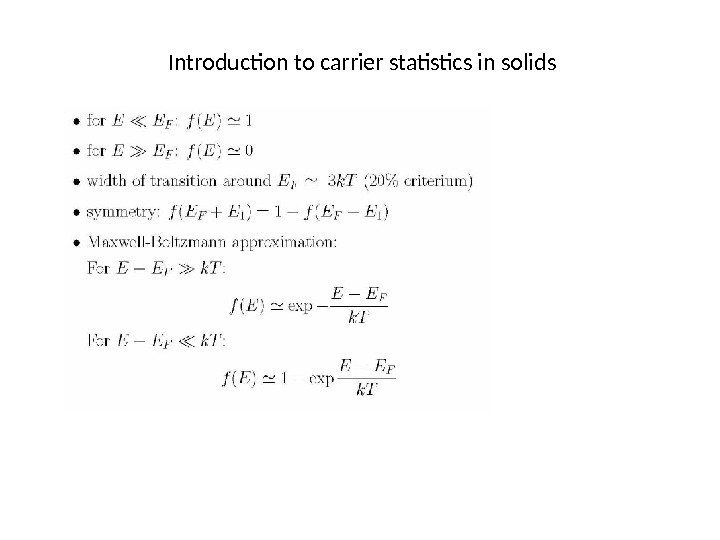Introduction to carrier statistics in solids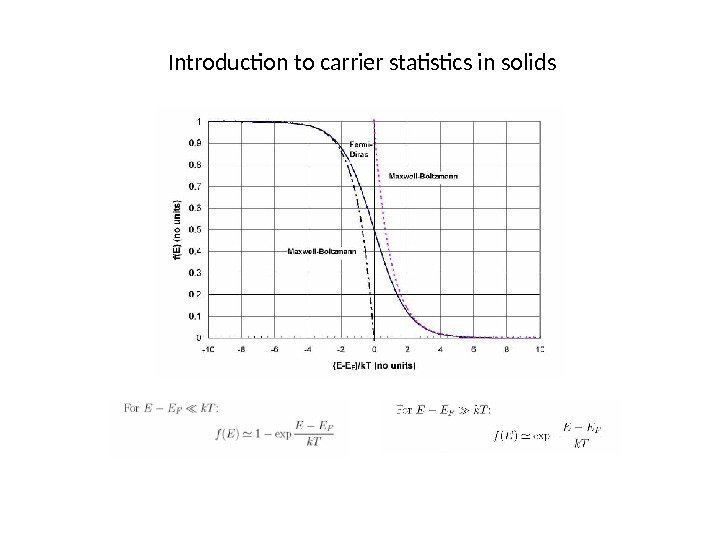Introduction to carrier statistics in solids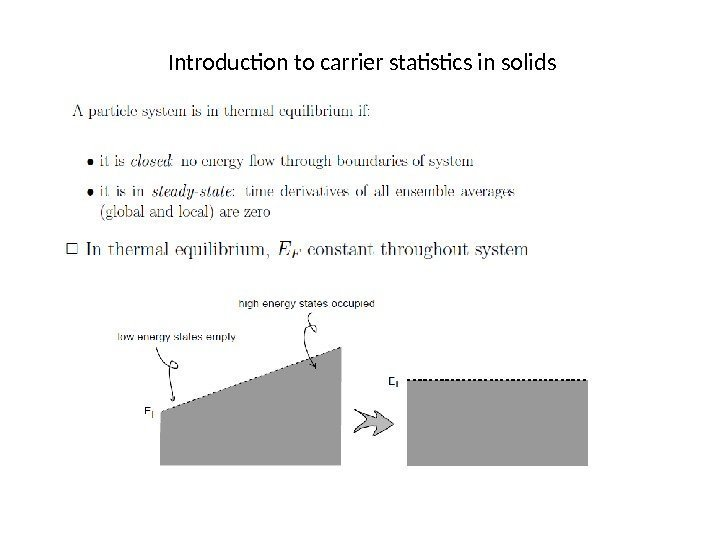Introduction to carrier statistics in solids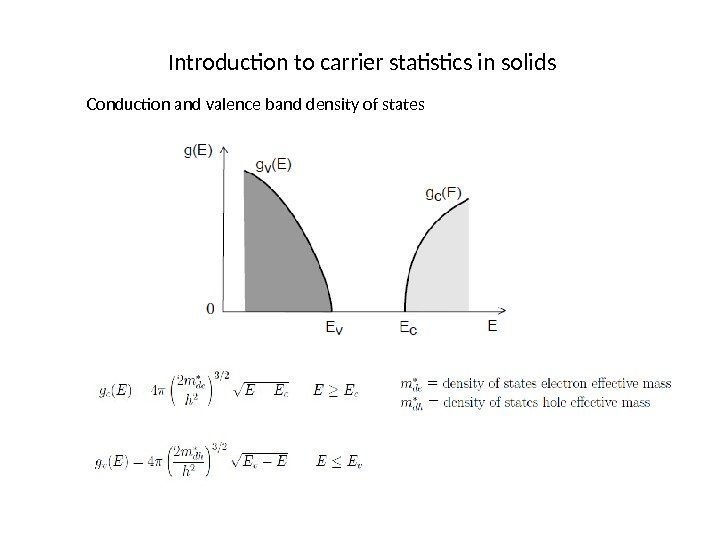Introduction to carrier statistics in solids Conduction and valence band density of states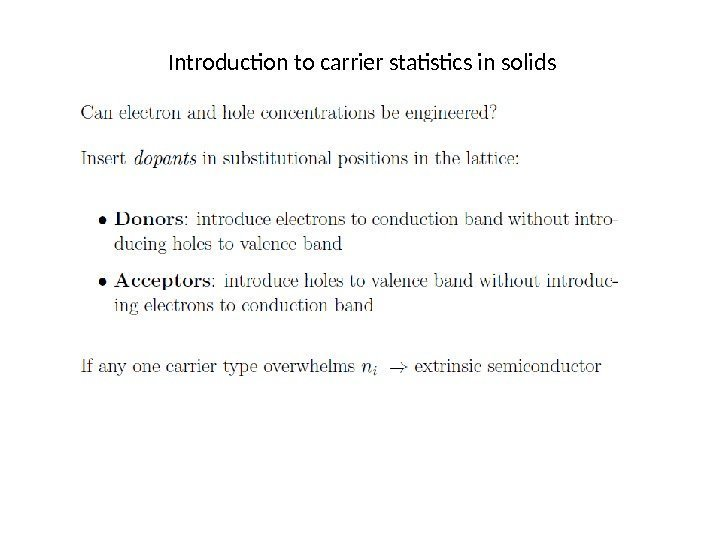Introduction to carrier statistics in solids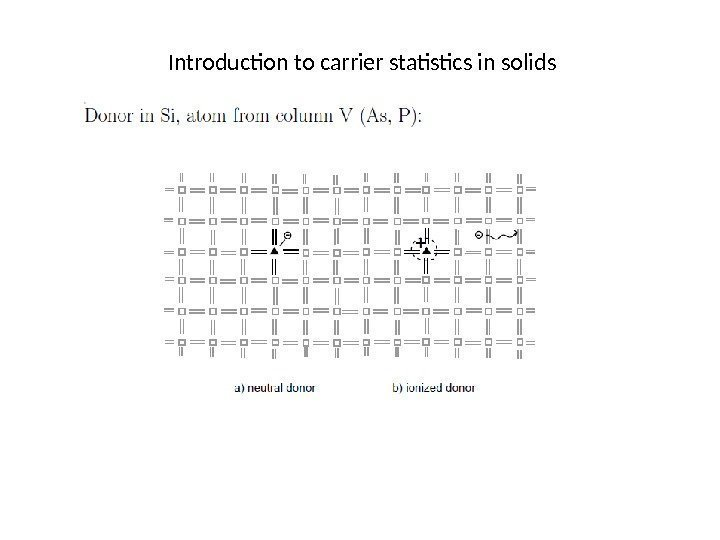Introduction to carrier statistics in solids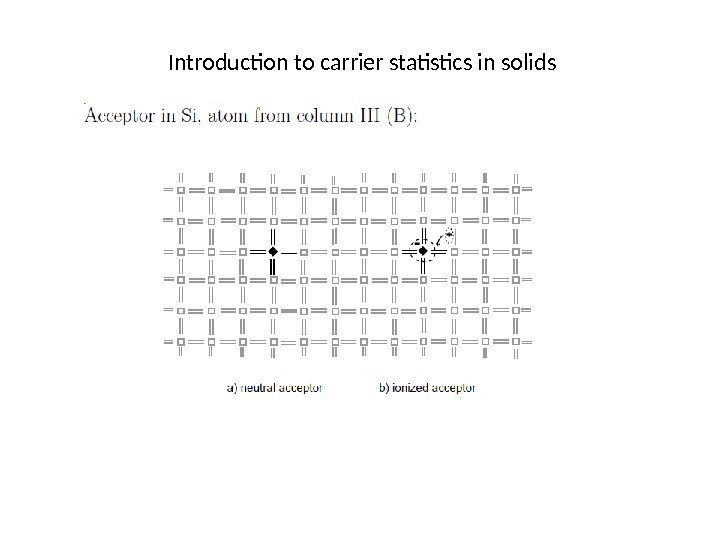Introduction to carrier statistics in solids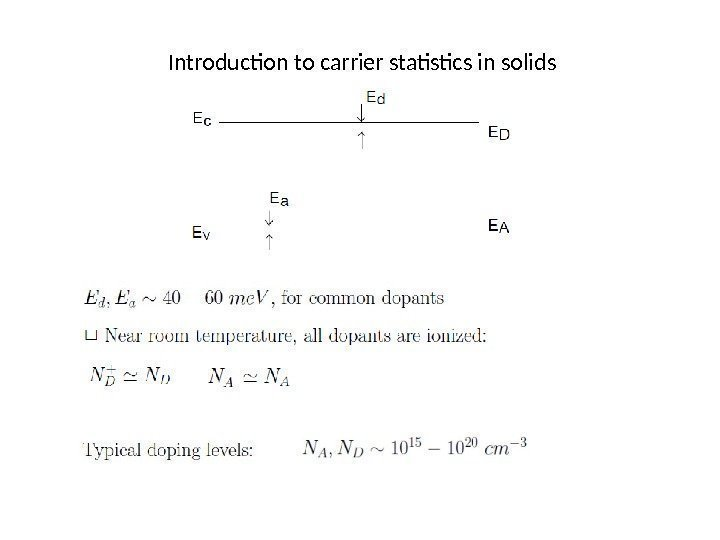Introduction to carrier statistics in solids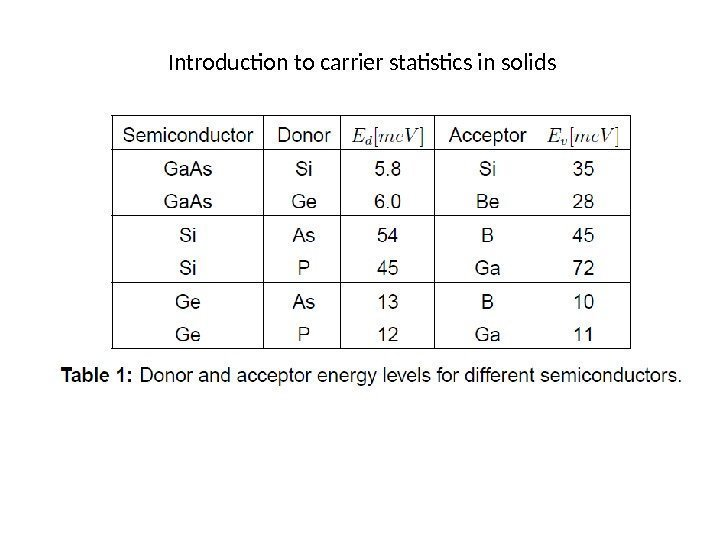Introduction to carrier statistics in solids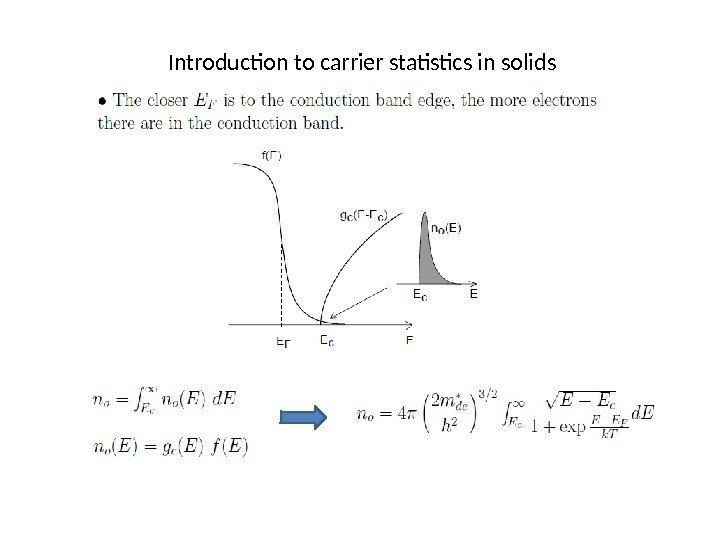Introduction to carrier statistics in solids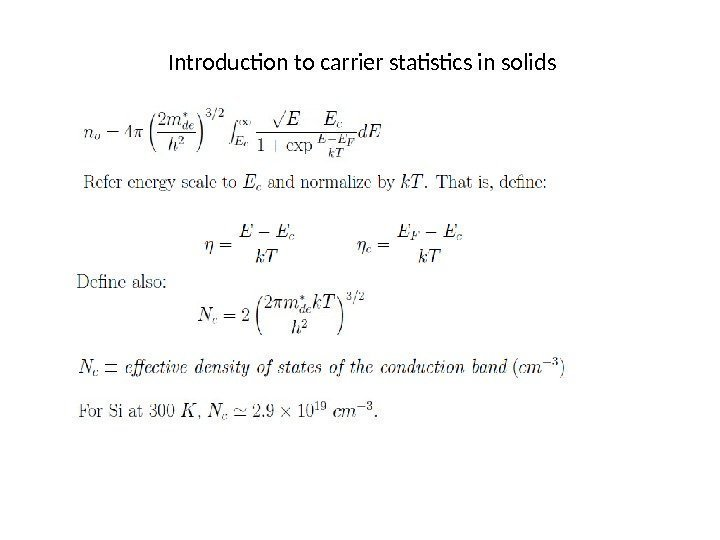Introduction to carrier statistics in solids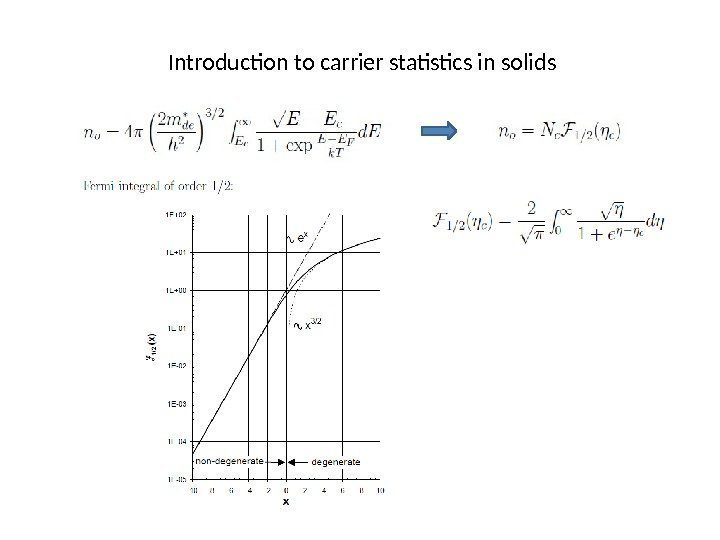Introduction to carrier statistics in solids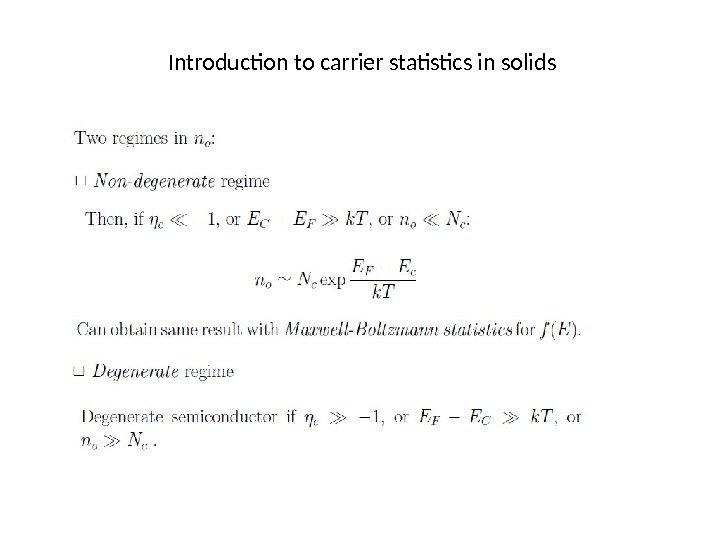Introduction to carrier statistics in solids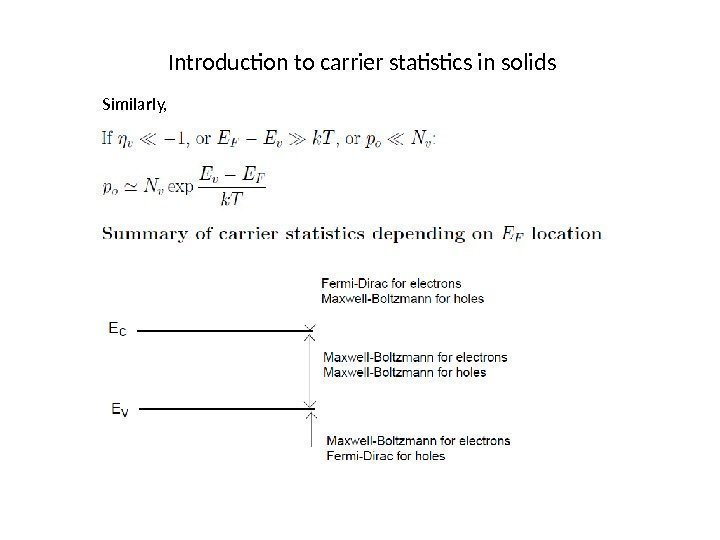Introduction to carrier statistics in solids Similarly,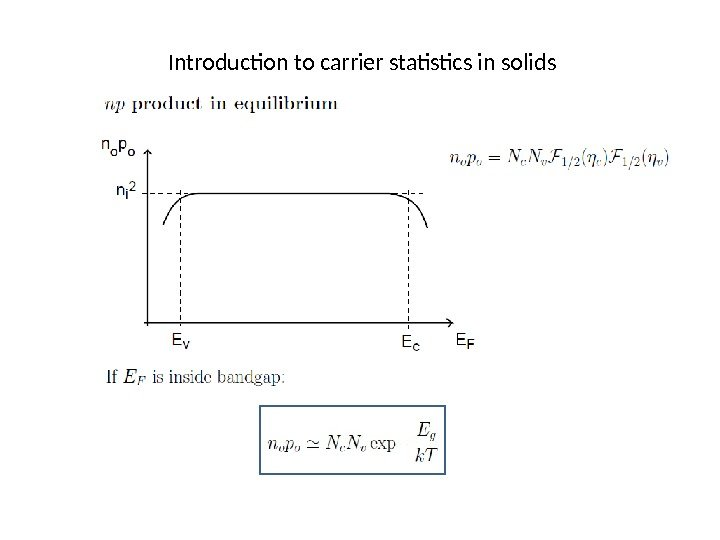Introduction to carrier statistics in solids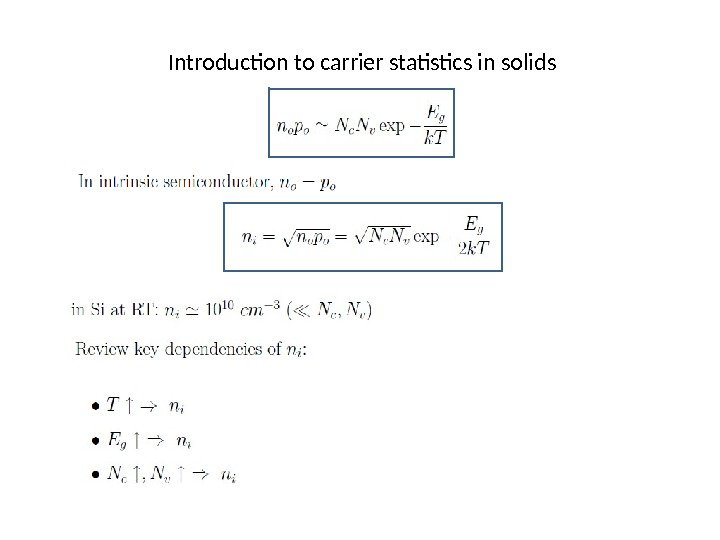Introduction to carrier statistics in solids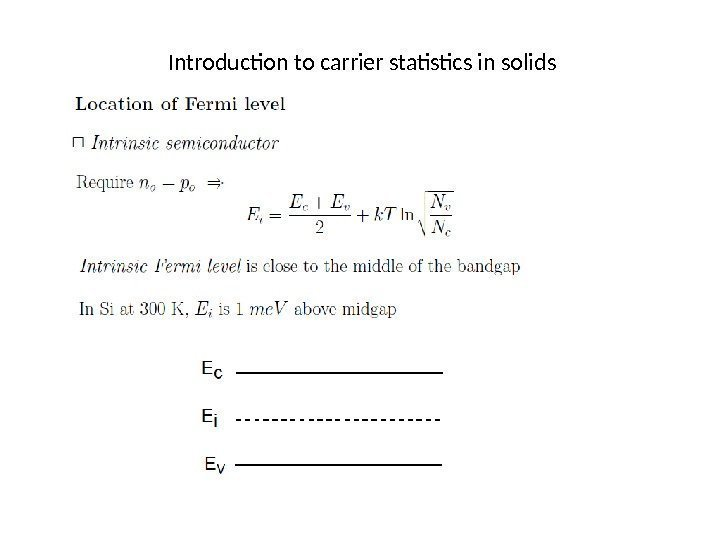Introduction to carrier statistics in solids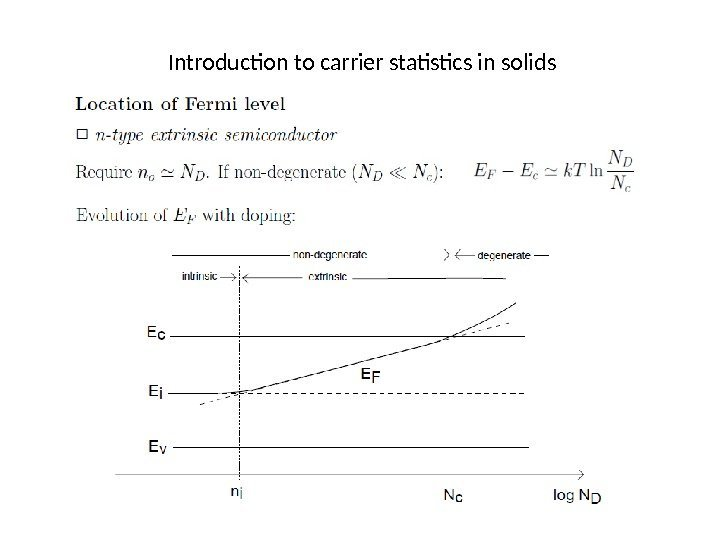Introduction to carrier statistics in solids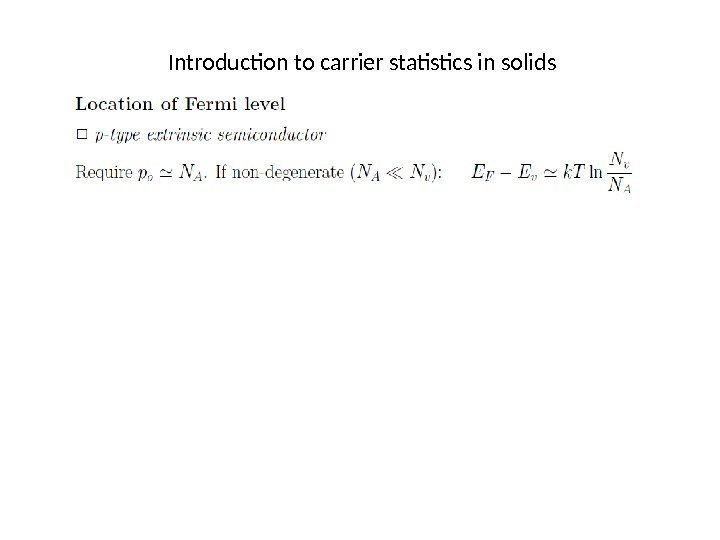Introduction to carrier statistics in solids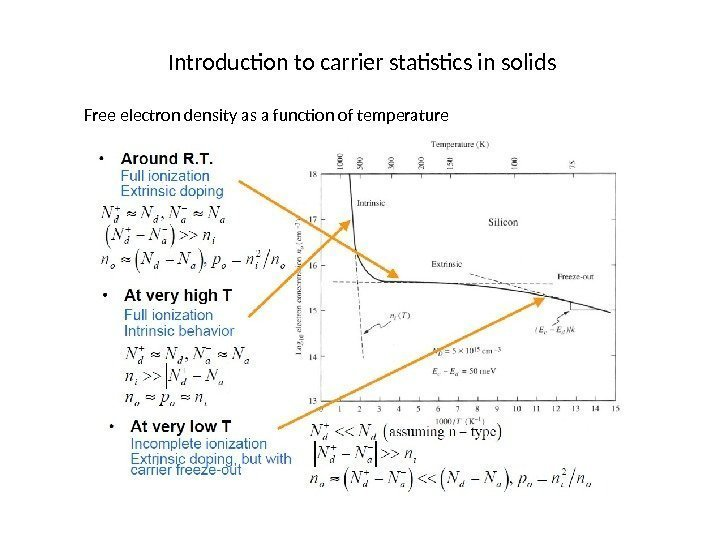Introduction to carrier statistics in solids Free electron density as a function of temperature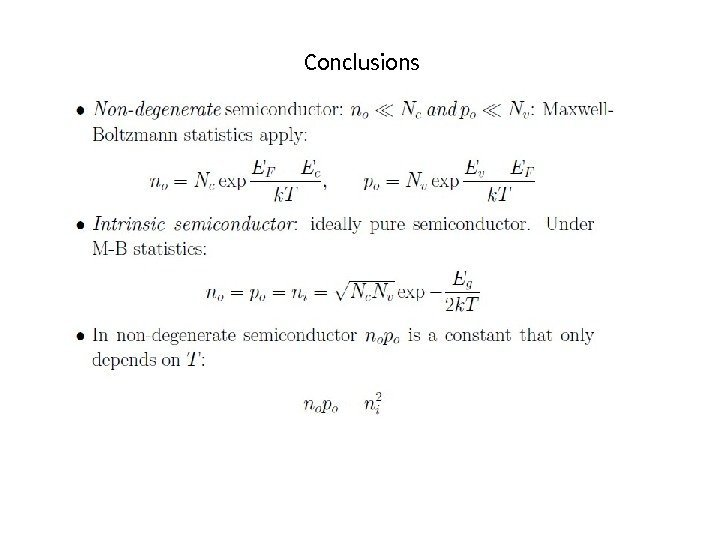Conclusions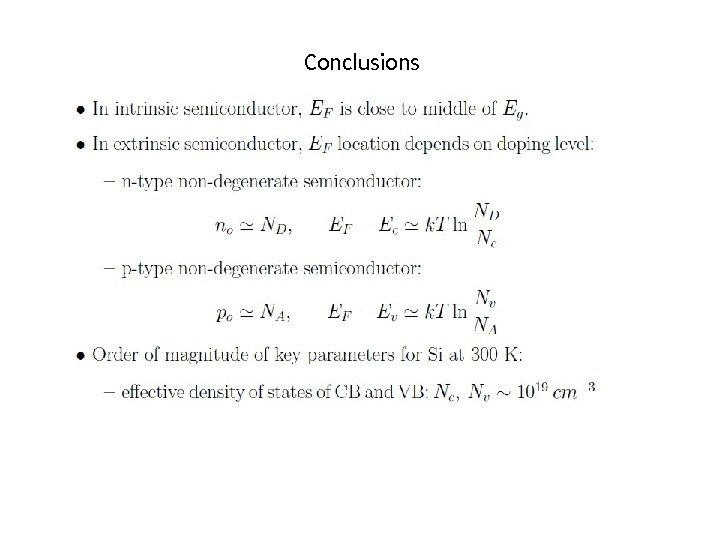Conclusions